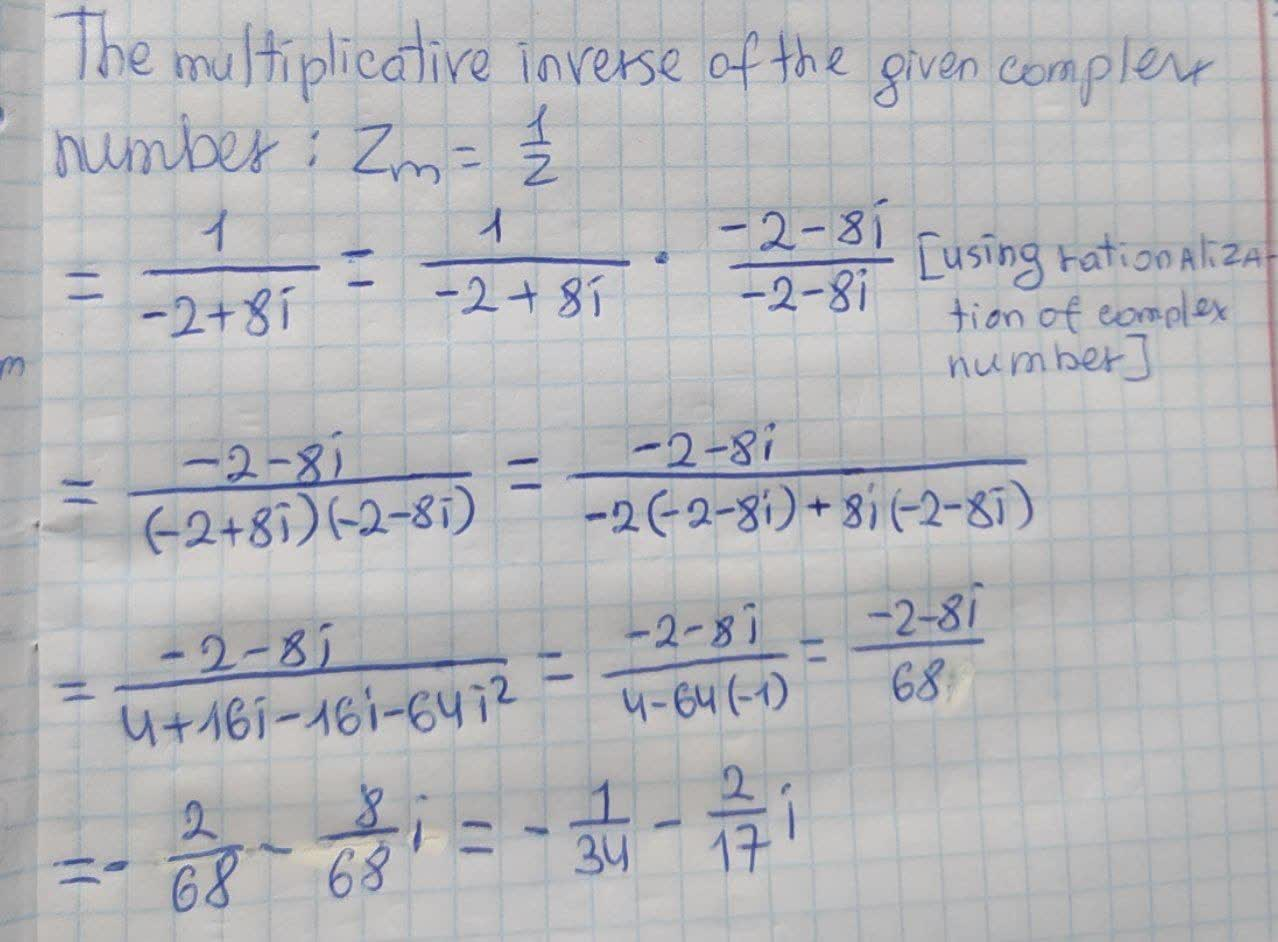Question# Multiplicative Inverse of a Complex Number The multiplicative inverse of a

Complex numbers
ANSWEREDMultiplicative Inverse of a Complex Number The multiplicative inverse of a complex number z is a complex number zm such that $$\displaystyle{z}\times{z}{m}={1}$$. Find the multiplicative inverse of complex number.
$$\displaystyle{z}=−{2}+{8}{i}$$